### Home > CALC > Chapter 1 > Lesson 1.2.6 > Problem1-95

1-95.
1. Use what you know about functions to quickly sketch the following. Then, check your answer on your graphing calculator. Homework Help ✎

1. y = (x − 2)2 + l

2.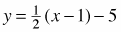3.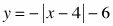4. y = 2x + l + 3

5. Find the domain and range of the function in part (d). Then find the domain and range of its inverse.

6. Which of the functions above have inverses that are not functions? Explain how you know.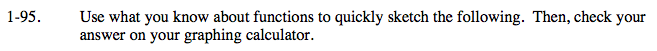First think about the parent graph. Then shift and stretch accordingly. Remember, asymptotes and holes will move with the graph.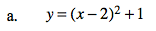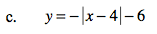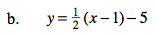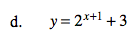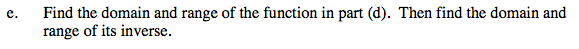The inputs and outputs of inverse functions switch. In other words, the domain and range switch.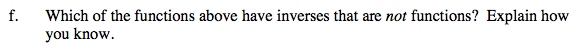Recall that a function can have only one output of each input.